# EE GATE 2015 PAPER 02 - Online Test

Q1. Choose the statement where underlined word is used correctly.
Explaination / Solution:
No Explaination.

Q2. Out of the following four sentences, select the most suitable sentence with respect to grammer and usage:
Explaination / Solution:
No Explaination.

Q3. Match the following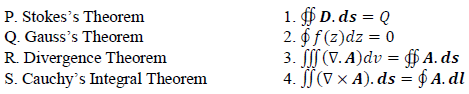Explaination / Solution:
No Explaination.

Q4.
The filters F1 and F2 having characteristics as shown in Figures (a) and (b) are connected as shown in Figure (c).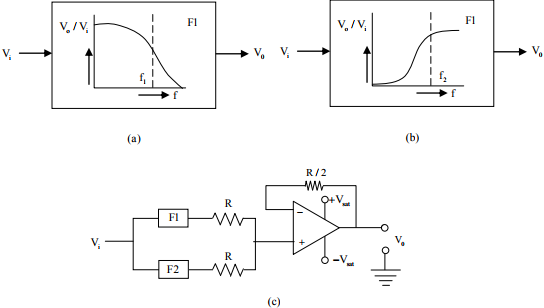The cut-off frequencies of F1 and F2 are f1 and f2 respectively. If f1 < fthe resultant circuit exhibits the characteristics of a

Explaination / Solution:
No Explaination.

Q5. The saturation voltage of the ideal op-amp shown below is ±10V. The output voltage v0 of the following circuit in the steady-state isExplaination / Solution: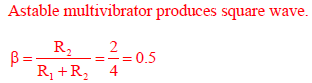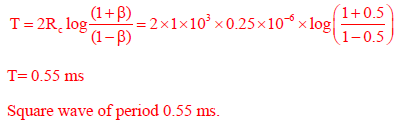Q6. A generic term that includes various items of clothing such as a skirt, a pair of trousers and a shirt as
Explaination / Solution:
No Explaination.

Q7. Two semi-infinite dielectric regions are separated by a plane boundary at y=0. The dielectric constant of region 1 (y<0) and region 2 (y>0) are 2 and 5, Region 1 has uniform electric field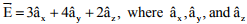are unit vectors along the x, y and z axes, respectively. The electric field region 2 is
Explaination / Solution:Q8. We ____________ our friend’s birthday and we ___________ how to make it up to him.
Explaination / Solution:
No Explaination.

Q9. Match the following:
Instrument Type                                                                        Used for
P. Permanent magnet moving coil                                            1. DC only
Q. Moving iron connected through current transformer            2. AC only
R. Rectifier                                                                                3.AC and DC
S. Electrodynamometer
Explaination / Solution:
No Explaination.

Q10. A 3-phase balanced load which has a power factor of 0.707 is connected to balanced supply. The power consumed by the load is 5kW. The power is measured by the two wattmeter method. The readings of the two wattmeters are
Explaination / Solution:satisfiying only for(A)